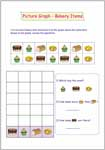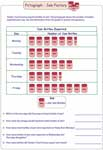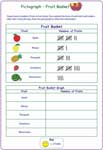Pictograph Worksheets

Picture graph worksheets contain cut-paste activity and reading graph. Students should answer the questions based on the picture graphs. Picture worksheets contain skills involving reading, drawing, grouping and more. A unique theme is maintained in each worksheet, which would help students to reinforce the knowledge in analyzing the data under real-life situations.

Picture Graph

Completing Picture Graph

Complete the picture graph by cutting out the graphics and pasting them under the suitable category. Based on the graph, answer the questions. Suitable for grade 1 and grade 2 students.Answer the questions based on the picture graph. Each worksheet has a different theme.Easy Level

It contains basic reading pictograph worksheets. Use the information to answer the questions. Keys: 2, 8 or 10.Moderate Level

The worksheets have half symbols with key varying in 2-digit numbers.Difficult Level

The scales are in hundreds. There are three worksheets in different themes. Calculation may be a bit heavier. Obviously, there are half symbols.Drawing Pictograph

Tally Marks and Pictograph

Draw the pictograph based on the information on tally marks. Scale varies between 2 to 10. You do not require half symbols here.Counting and Grouping

Draw the pictograph to show the count of each item. Students may group the items in 2s, 3s or 5s.Standard: Easy Level

Draw pictograph to represent the data and answer the questions. Key value varies between 2 and 9.Standard: Moderate Level

Each worksheet has real-life representation with data values in the table. Show the information by drawing the pictograph. Students may use half-symbols in some places.Standard: Difficult Level

Divide each entry by key value to determine the number of symbols that go in each row. Key values take only three-digit numbers. Use half-symbols wherever necessary.Stock up your library with the complete set of worksheets on pictograph.

Related Worksheets